Steen Magnussen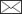An assessment of three variance estimators for the k-nearest neighbour technique

Magnussen S. (2013). An assessment of three variance estimators for the k-nearest neighbour technique. Silva Fennica vol. 47 no. 1 article id 925. https://doi.org/10.14214/sf.925

Abstract

A jackknife (JK), a bootstrap (BOOT), and an empirical difference estimator (EDE) of totals and variance were assessed in simulated sampling from three artificial but realistic complex multivariate populations (N = 8000 elements) organized in clusters of four elements. Intra-cluster correlations of the target variables (Y) varied from 0.03 to 0.26. Time-saving implementations of JK and BOOT are detailed. In simple random sampling (SRS), bias in totals was ≤ 0.4% for the two largest sample sizes (n = 200, 300), but slightly larger for n = 50, and 100. In cluster sampling (CLU) bias was typically 0.1% higher and more variable. The lowest overall bias was in EDE. In both SRS and CLU, JK estimates of standard error were slightly (3%) too high, while the bootstrap estimates in both SRS and CLU were too low (8%). Estimates of error suggested a trend in EDE toward an overestimation with increasing sample size. Calculated 95% confidence intervals achieved a coverage that in most cases was fairly close (± 2%) to the nominal level. For estimation of a population total the EDE estimator appears to be slightly better than the JK estimator.

Author Info

Received 26 July 2012 Accepted 14 February 2013 Published 11 June 2013

Views 92538Supplementary Files
xyCLUPOP1[CSV]
xyCLUPOP2[CSV]
xyCLUPOP3[CSV]

# 1 Introduction

The k-nearest neighbour technique (kNN) is used to impute values of one or more target variables (Y) for elements in a finite population without a direct observation of Y (Paass 1985; Aha 1997). Imputations are based on a set of selected auxiliary variables (X) known for all (N) elements in the population and correlated with Y. In a typical kNN application, a probability sample of n elements provides paired observations of X and Y. A population element can be a pixel in an image of the population or simply a fixed-sized contiguous regular spatial area suitable for a tessellation of the population and compatible with the scale of the target variable.

The sample of n elements is referred to as the reference set, while the N–n elements with no observation of Y, are referred to as the target set (Tomppo 1991). The imputed Y-value for an element in the target set is a fixed known function (f) of the k Y-values in the reference set whose associated X-values are closest – in terms of a selected distance metric – to the X-values in the element to receive an imputation. The analyst chooses X, f, k, and the distance metric; usually through a combination of cross-validation procedures and ranking of goodness-of-fit statistics (McRoberts 2009).

The appeal of the kNN technique is: i) simple and flexible (non-parametric, distribution-free), ii) predictions of the target variable(s) for all elements in a population (suitable for mapping), iii) small area estimation of totals, and iv) an ability to handle multivariate imputations as easily as univariate imputations (Crookston and Finley 2008). The most important detractors are: i) difficulty in optimizing performance (bias and accuracy); ii) the curse of dimensionality, and iii) a lack of small area estimators of variance.

Leave-one-out cross-validation is often used to guide the analyst towards a good choice of k, an appropriate function f, and a suitable distance metric. Selection of X-variables have been done in the context of regression modeling or with more advanced methods like simulated annealing and genetic algorithms (Tomppo and Halme 2004; Barth et al. 2009). Given the non-parametric (distribution-free) nature of kNN, and the lack of a proper joint distribution of imputations (Lin and Jeon 2006), one cannot infer performance at the population level from in-sample point estimates of bias and accuracy (Chen and Shao 2000; Baffetta et al. 2009).

The curse of dimensionality (Scott 1992, p. 27) manifests itself by the fact that distances in X-space from a target element to the n reference elements become increasingly similar with the dimension of X. Conversely, the number of reference elements with a similar distance to a target element grows exponentially with the dimension of X. Or, as stated by Beyer et al. (1999), “when dimensionality increases, the distance to the nearest data point approaches the distance to the farthest data point”.

In forestry the kNN technique has appeal (Maltamo and Kangas 1998; Holmström and Fransson 2003; Maselli et al. 2005; LeMay et al. 2008; Breidenbach et al. 2010) due to: i) readily available low-cost remotely-sensed auxiliary variables correlated with Y; and ii) difficulties encountered with alternative parametric and non-parametric multivariate modeling approaches (Koistinen et al. 2008) due to locally varying relationships between X and Y in response to variation in species, ages, forest structures, soils, and climate (Zhang and Shi 2004; Opsomer et al. 2008; McRoberts et al. 2010), and iii) predictions of individual population elements for mapping and small area estimation problems (Tomppo 2006).

A summary of a kNN inventory typically requires an estimate of precision of estimated strata and population totals. In early kNN applications, an estimate of precision was typically given as the average, over the n-reference elements, root-mean-squared error (RMSE) obtained in a leave-one-out cross-validation (e.g. Maltamo and Kangas 1998; Katila and Tomppo 2001). However, the RMSE only applies to the sampled elements and cannot be scaled to a population (stratum) total (Kim and Tomppo 2006; McRoberts et al. 2007). Kim and Tomppo (2006) proposed an ordinary kriging based estimator of the variance of element-level predictions (Cressie 1993, p. 127) and block-kriging (Van der Meer 2012) for small area estimation problems (SAE). However, the underlying assumption of stationarity is rarely reasonable in forestry. As well, the application of ordinary kriging theory to a prediction based on the k-nearest neighbours and not a weighted sum of all n reference elements may lead to a bias, akin to the bias following from a tapering of a covariance matrix (Kaufman et al. 2008).

McRoberts et. al (2007) was the first to propose a kNN variance estimator for a population total that explicitly considered the variance and covariance of all population elements in both simple random and cluster sampling designs. I refer to this estimator as ‘vMCR’. Computation of vMCR involves a double-summation over all elements included in a total. There was no formal testing of vMCR but in three case studies it agreed well with bootstrapped estimates of variance (McRoberts et al. 2011). Computation time for vMCR can be sharply reduced by a Monte Carlo approximation to the double summation (McRoberts et al. 2011). vMCR can serve as a direct estimator of variance in SAE problems (Särndal et al. 1992).

Magnussen et al. (2009) proposed a model-based mean squared error (MSE) estimator for a kNN estimate of a population mean. They showed that the average element-level MSE estimator from a leave-one-out cross-validation underestimates the MSE of an areal mean by a significant margin. The applicability of their MSE to SAE problems requires further investigation. Chen and Shao (2000) developed a design-based estimator of variance for nearest neighbour imputations (k = 1), but it is only applicable to imputation of missing values in a planned survey.

Baffetta et al. (2009) proposed a design-based “empirical-difference” variance estimator (vEDE) for a bias-corrected kNN estimator of a population total (mean). They viewed kNN imputations as proxy values for the target variable (Särndal et al. 1992, p. 221). Simulated simple random sampling from a population of N = 312 and relative sample sizes of 5%, 10%, 20%, and 30%, illustrated that the estimated relative bias of vEDE declined with increasing sample size. Bias became unimportant (< 5%) for the three largest sample sizes with k > 3 and when the coefficient of determination between Y and X was less than 0.8. By construct EDE and vEDE are limited to direct estimation of totals and variances in SAE problems.

An alternative design-based resampling variance estimator was proposed by Magnussen et al. (2009). A modified balanced repeated replication (BRR) scheme (Wolter 2007, p. 117) was employed to obtain estimates of element-level variances and covariances and a variance estimator of a total (vBRR). A comparison of vEDE and vBRR in simulated sampling from seven populations (576 ≤ N ≤ 900) indicated a good performance of both estimators in simple random sampling (SRS) and one-stage cluster sampling (CLU). In theory vBRR is suited for design-based inference in SAE problems (Särndal et al. 1992).

Nothdurft et al. (2009) were among the first to use a bootstrap resampling variance estimator in a forest inventory kNN application (k = 1). Their primary objective was to compare variability in stand-level kNN imputations and in calibrated kNN empirical best linear unbiased predictors (EBLUP).

In a recent study of actual forest inventory kNN applications, McRoberts et al. (2011) compared vMCR to a jackknife (vJK) and a bootstrap (vBOOT) estimator of variance. In the case of SRS the differences between vMCR0.5, vJK0.5, and vBOOT0.5 were minor (< 3 %). For CLU the agreement between vMCR and vBOOT (no vJK results for clustered data) was generally satisfactory, but less so than in the SRS designs. An unexplained systematic effect of the bootstrap resampling protocol applied to clustered data (single-stage cluster sampling versus single-stage cluster sampling followed by simple random sampling within clusters (Field and Welsh 2007)) was manifest. With large clusters (14–18 elements) the two-stage bootstrap sampling generated vBOOT estimators that agreed with vMCR estimators. With small clusters the agreement was lacking. Firm conclusions cannot be drawn from these non-replicated case studies. The application of vJK and vBOOT to SAE problems requires further study.

The current lack of an extensive testing of kNN variance estimators for a population total, and sheer absence of tested kNN variance estimators for SAE problems, makes it difficult to make recommendations to practice. As a first step towards improving the situation, this study compares the performance (bias, accuracy, and coverage of calculated nominal 95% confidence intervals) of three variance estimators for a kNN estimate of a population total: vJK, vBOOT, and vEDE in simulated sampling from three artificial yet realistically complex multivariate populations (N = 8000). Results are presented for four sample sizes in SRS and CLU. vBRR was not included because it is complex and still demands too much computer time to be of practical use. SAE problems are beyond the scope of this study. To qualify as a suitable kNN variance estimator in SAE problems an estimator must first qualify as suited at the population level. A follow-up study of potentially suitable kNN variance estimators for SAE problems is anticipated.

In forestry, population sizes are generally large (N ≥ 104), and sample fractions are low (n / N ≤ 0.01), yet the number of reference elements is relatively large (n > 100). Hence, resampling estimators of variance for a kNN estimate of a population total can be computationally demanding despite a steady increase in the processing speed of desk-top computers. Fast neighbour search algorithms like the kd-tree’ (e.g. Finley et al. 2006) in, for examples, the R-package ‘yaImpute’ (Crookston and Finley 2008), MATLAB®, and MATHEMATICA (Wolfram 1999), and parallel processing has sharply reduced the problem. Computations with graphics processor units (GPUs) will eventually deflate the time issue (Schenk et al. 2008). To improve the practicality of resampling estimators of variance for a population total, this study details steps that will curtail the time needed to compute a vJK or a vBOOT estimate.

# 2 Material and methods

## 2.1 Population, sampling objectives, and notation

A finite area population U composed of N equal-area spatial elements is considered with the objective of estimating the total of one or more target variables (Y) from a probability sample of size n. A set of auxiliary variables (X) is known for every element in the population. The auxiliary variables have been selected on grounds of their ability to predict Y. The probability sample (s) is obtained, without replacement, by either SRS or CLU. The population contains M clusters of size m so that N = m × M with m = 1 in SRS. In SRS a sample unit is equal to a population element; in CLU it is a cluster of m elements. Notation is for a univariate Y but extension to a multivariate case requires no new theory.

## 2.2 The kNN estimator

The kNN estimator of Y in the ith population element (Haara et al. 1997) can be written succinctly as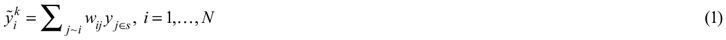where summation in Eq. 1 is over the k elements (j ~ i) in the sample (s) with auxiliary variable values closest to Xi, and wij is the weight given to the reference element yj∈sj~iwij=1). Euclidean distances in X-space were used for the selection of the k-nearest neighbours. A standardized Euclidean distance metric is used throughout; i.e. the X-variables have been standardized to a mean of zero and a variance of one for the computation of distances in X-space. This is also the metric used by the ‘euclidean’ option in the R-package ‘yaImpute’ (Crookston and Finley 2008).

In practice the weights may be a function of distance that optimizes precision (McRoberts 2009). Here wij = k–1 since the choice of weights is inconsequential for an assessment of the kNN variance estimators.

A kNN estimator of the population total of Y (Ty) is denoted asand computed as the sum of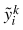over the N population elements. The expected value ofandover all possible samples are invariant to the sampling design (Baffetta et al. 2009).

## 2.3 The jackknife kNN estimator

The jackknife kNN estimator (JK) of Ty (Efron 1982) iswhere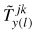is the kNN estimator (pseudo-value) of Ty after deleting the lth sample unit (l = 1,…,n). The jackknife estimator of variance (vJK) of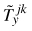is then (Wolter 2007, p. 153)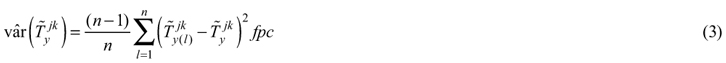where fpc is the finite population correction factor 1 – n × m / N. The variance estimator vJK is used as an estimator of the variance of(Wolter 2007, p. 153). Appendix A provides a time-saving implementation of the jackknife estimators.

## 2.4 The bootstrap kNN estimator

The bootstrap kNN estimator of Y in the ith population element (yi) and bth bootstrap sample iswhere s*(b) is the bth bootstrap sample generated by SRS with replacement from the original sample of n sample units (Field and Welsh 2007). Accordingly, a bootstrap replication estimator of a population total is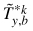whereis the sum of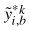over the N population elements. The bootstrap estimator (BOOT) of Ty is the mean of the B bootstrap replication estimates, here denoted as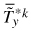. It follows that the bootstrap estimator of variance (vBOOT) of is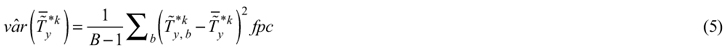As in the case of vJK, the vBOOT in Eq 5 will be used as a variance estimator for(Wolter 2007, p. 195).

In implementations of the bootstrap procedure, a replicate (b) specific N × k array of nearest neighbour identifiers (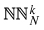(b), see Appendix A for details) is needed for each bootstrap sample, making computing times for vBOOT B times longer than for. A faster bootstrap variant of BOOT (called FBOOT) is detailed in Appendix A. Variances estimated with this variant are denoted vFBOOT.

## 2.5 The empirical difference estimator

Baffetta et al. (2009) proposed a design-based bias-adjusted empirical difference estimator (EDE) of Ty for element sampling (m = 1). With an extension to clusters of size m ≥ 1, the EDE becomes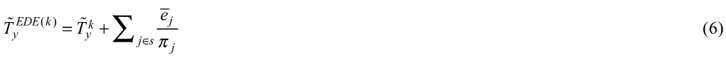where πj is the sample inclusion probability of sample unit j (Särndal et al. 1992), and e̅j is the mean of the m differences between the actual and the kNN imputed values of Y in the jth sample unit (j = 1,…, n). In SRS m = 1 and e̅j is simply the difference (residual) for the jth sample unit. The associated Horvitz-Thompson type estimator of variance (vEDE) iswhere πjh is the joint sample inclusion probability of sample units j and h.

## 2.6 Estimator performance

The kNN, JK, BOOT, FBOOT, and EDE estimators of Ty are assessed for bias in simulated SRS (m = 1) and CLU (m = 4) from three artificial yet realistic populations (see 2.8). Bias is estimated as the difference between the mean of 400 replicated kNN estimates of a total and the known true total (see 2.7). For ease of comparison the bias is expressed in percent of a true total.

Variance estimates obtained with vJK, vBOOT, vFBOOT, and vEDE were compared to the corresponding empirical estimate of variance (vEMP) of replicated estimates of a kNN total (see 2.7). Normal quantile 95% confidence intervals for estimated population totals, calculated from vJK and vEDE estimates of variance, are assessed for their coverage (relative frequency with which a calculated confidence interval includes Ty). Accelerated and bias-corrected normal quantiles were used to estimate coverage of bootstrap intervals (Efron and Tibshirani 1993, p. 188).

The distributions of replicated estimates of a total (see 2.7) were tested for normality at the 5% level with an Anderson-Darling (AD) test (Anderson and Darling 1952), and differences between estimated and actual totals (bias) were tested under the null hypothesis of a zero mean normal distribution (AD test). Distributions of estimated JK, BOOT, FBOOT, and EDE totals were tested for equality to the distribution of(AD test).

The replicate mean of vJK, vBOOT, vFBOOT, and vEDE estimates of variance was compared to vEMP and tested for equality with an AD test using a bootstrap distribution of 400 paired differences.

## 2.7 Sampling designs

Sample size in SRS was n = 50, 100, 200, and 300 (m = 1), and in CLU sampling n = 20, 30, 50, and 100 (m = 4). With a population size (N) of 8000 (see 2.8) the sample fractions for SRS were: 0.00625, 0.0125, 0.025, and 0.0375. Under CLU they were: 0.01, 0.015, 0.025, and 0.05. The number of nearest neighbours (k) tested was k = 1, 2, 4, 6, 8, 10, and 12. Each of the 2 (m) × 4 (n) × 7 (k) = 56 designs was replicated nrep = 400 times followed by a computation of estimator specific kNN totals and variances. The number of bootstrap replications (B) within each of the 400 replications was fixed at 60. A study of Monte-Carlo errors (Koehler et al. 2009) suggested that 400 replications sufficed to declare two distributions of estimated variances significantly different at the 5% level when their means differed by 10% or more (AD test).

## 2.8 Case studies

Three artificial multivariate populations (POP1, POP2, and POP3) of size N = 8000 elements were generated from known marginal distributions of X and Y and a target correlation coefficient between the variables in X and Y.

There are three Y-variables (Y1, Y2, and Y3) in each population, three X-variables in POP1 (X1, X2, and X3), and four in POP2 and POP3 (X1, X2, X3, and X4). The marginal distributions of variables in the three populations were complex in order to reflect scenarios with skewed, multi-modal, and non-Gaussian distributions in forest inventory applications as seen in actual inventory data (LeMay et al. 2008; Magnussen et al. 2009). Details of the populations are in Appendix B. The data used in this study are available as supplementary files.

It is recognized that the size of the simulated populations are orders of magnitude smaller than in practical kNN applications (Katila 2006; Bernier et al. 2010). Yet, the bias of a kNN estimator appears to be largely a function of sample size, and not population size (Katila 2006; McRoberts et al. 2011). A doubling of N would have added just 1% to the marginal variance of the target variables. A further doubling would have added less than 0.5% to the variances. Finally, the accuracy of the tested variance estimators is governed by intrinsic properties and sample sizes more than population size and sample fractions (Särndal et al. 1992). On an absolute scale, the studied sample sizes – like those in practice ‒ are small and should vouch for the practical relevance of the study.

# 3 Results

## 3.1 Choice of k

The k-value that resulted in the lowest RMSE varied from a low of 4 in POP1 to a high of 8 (POP2 with SRS and POP3 with CLU). The intermediate value of 6 was best in POP2 with CLU and POP3 with SRS. However, the effect of k on RMSE was modest once k was equal to or greater than 4. A common choice of k = 6 would not have changed the results by much.

Henceforth results are only reported for the k-value for which the average (across Y-variables) relative RMSE (in percent of the actual total) of a kNN totalwas lowest.

## 3.2 Bias

Under a SRS design the kNN estimator of a total had a bias ≤ 0.3% in POP1 and POP3 (Table 1). In POP2 bias approached 1% for Y1 and the two smallest sample sizes (50, 100). Apart from these two cases, there was no apparent effect of sample size. Results for JK, BOOT and FBOOT were almost identical. Bias for non-reported k-values were not materially different. Bias ofwas in 7 out of 10 cases less than the bias of. On average, EDE reduced the bias inby approximately 33%; in just two cases (out of 36) did EDE results suggest a slightly larger bias than in.

 Table 1. Relative bias (RB%) of kNN and EDE estimators of totals in SRS (m = 1). RB% = (estimate – actual) / actual × 100. Results are for the k-value (kopt) that minimized the RMSE of an estimated total. POP (kopt) n kNN EDE Y1 Y2 Y3 Y1 Y2 Y3 POP1 (4) 50 –0.1 0.2 0.2 –0.0 0.0 0.2 100 –0.3 –0.1 –0.4 0.1 –0.2 –0.3 200 –0.4 –0.1 –0.3 0.0 0.0 0.3 300 –0.3 0.1 –0.2 0.1 0.1 –0.2 POP2 (8) 50 –0.8 –0.2 –0.4 0.3 0.4 0.4 100 –0.6 0.3 –0.5 –0.3 0.1 0.2 200 –0.2 0.1 –0.4 0.1 0.2 –0.0 300 –0.1 0.2 –0.3 0.1 0.1 0.1 POP3 (6) 50 0.3 0.2 0.2 0.3 0.1 0.1 100 0.5 0.3 0.0 0.4 0.3 0.2 200 –0.4 –0.2 0.3 –0.1 0.0 0.2 300 –0.4 0.0 0.3 0.1 0.1 –0.2
 Table 2. Relative bias (RB%) of kNN and EDE estimators of totals in CLU (m = 4). RB% = (estimate – actual) / actual × 100. Results are for the k-value (kopt) that minimized the RMSE of an estimated total. POP (kopt) n kNN EDE Y1 Y2 Y3 Y1 Y2 Y3 POP1 (4) 20 –0.0 0.2 0.5 0.5 0.1 0.6 30 –1.0 –0.2 –0.5 –0.6 –0.2 –0.6 50 –0.5 0.1 –0.3 –0.1 –0.3 –0.1 100 –0.4 –0.2 –0.1 –0.1 –0.2 –0.1 POP2 (6) 20 –0.4 0.6 –0.3 –0.1 0.6 0.2 30 –0.1 0.0 0.3 0.2 –0.1 –0.0 50 –0.2 –0.0 0.4 –0.0 –0.2 –0.0 100 –0.2 –0.0 –0.4 –0.1 –0.2 –0.1 POP3 (8) 20 –0.5 –0.6 0.4 –0.5 –0.7 0.2 30 –1.1 –1.0 0.9 –0.7 –0.9 0.3 50 –0.4 –0.2 0.6 –0.1 0.0 –0.1 100 –0.3 0.0 0.2 0.2 0.2 –0.5

Results for CLU followed trends seen in SRS except for a larger variation, and a tendency towards a larger bias for the two smallest sample sizes (Table 2). A bias between 0.5% and 1.0% was encountered in 9 out of 36 cases inand. In POP1 EDE was slightly less efficient in reducing bias than in POP2 and POP3. Fortunately, the squared bias was much smaller than the associated variance. Hence, inferences based on estimated variances will be approximately valid (Cochran 1977, p. 12).

The distribution of the 400 replicated estimates of a kNN totals were approximately normal in SRS designs (P-values in AD-tests were above 0.10 in 137 out of 144 cases). Under CLU there was no rejection of the hypotheses of a Gaussian sampling distribution of estimated totals.

## 3.3 Standard errors

The jackknifed estimates of standard error vJK0.5 were, in SRS, close to vEMP0.5 (Table 3), the difference was just 1% in half the scenarios. The hypotheses of equal variances (vJK = vEMP) were not rejected at the 5% level of significance. Yet distributions of standardized estimates of vJK were significantly different from a normal distribution in all but four cases.

SRS standard errors estimated from vBOOT were, on average, with n = 50 approximately 8% lower than vEMP0.5. Four of nine vBOOT0.5 with n = 50 were significantly smaller than vEMP0.5. For larger sample sizes, underestimation was approximately 3% but not significantly different from vEMP0.5.

SRS standard errors estimated from vFBOOT were generally smaller than vEMP0.5 estimates. With n = 50 the average underestimation reached 4%, and there were four cases with a statistically significant underestimation. For larger n the underestimation was, approximately 2%. The null hypotheses of no difference between vBOOT and vFBOOT were not rejected at the 5% level of significance. vFBOOT was 22 times out of 36 closer to vEMP than was vBOOT.

SRS standard errors obtained from vEDE were slightly conservative with an overestimation that increased with sample size from 3% with n = 50 to 7% with n = 300 (Table 3). However, only one estimate (POP1, Y1, n = 50) was found to be statistically significantly different from vEMP0.5.

 Table 3. Estimates of relative standard error (se%) in SRS. (se% = standard error of total ÷ total × 100). An estimate significantly different from its empirical counterpart at the 5% level (AD-test) is indicated in gray. Varianceestimator POP1 (kopt = 4) POP2 (kopt = 8) POP3 (kopt = 6) n = 50 100 200 300 50 100 200 300 50 100 200 300 vEMP Y1 9.7 6.4 4.2 3.4 6.4 4.5 3.1 2.2 8.0 5.1 3.6 2.9 Y2 9.2 6.0 4.0 3.4 7.0 4.4 3.2 2.5 8.0 5.5 3.7 3.0 Y3 9.8 6.9 5.0 3.8 5.8 3.7 2.8 2.1 9.5 6.2 4.2 3.3 vJK Y1 9.7 6.6 4.6 3.6 6.9 4.6 3.1 2.4 7.9 5.4 3.7 3.0 Y2 9.2 6.4 4.4 3.6 7.0 4.7 3.2 2.6 8.2 5.6 3.8 3.1 Y3 9.8 7.3 5.1 4.1 5.8 4.0 2.8 2.2 9.8 6.2 4.3 3.5 vBOOT Y1 8.7 5.9 4.1 3.3 6.5 4.3 2.9 2.3 7.3 5.0 3.5 2.8 Y2 8.4 5.8 4.0 3.2 6.5 4.5 3.0 2.4 7.6 5.1 3.6 2.9 Y3 9.5 6.6 4.6 3.7 6.4 3.7 2.6 2.1 8.1 5.7 4.0 3.2 vFBOOT Y1 8.8 6.1 4.2 3.4 6.1 4.2 2.9 2.3 7.3 5.1 3.5 2.8 Y2 8.8 6.0 4.2 3.4 6.4 4.5 3.0 2.4 7.6 5.2 3.6 2.9 Y3 9.7 6.7 4.8 3.8 6.1 3.7 2.6 2.1 8.2 5.8 4.1 3.3 vEDE Y1 9.5 6.6 4.6 3.7 6.7 4.5 3.1 2.5 7.8 5.4 3.8 3.1 Y2 9.3 6.4 4.5 3.7 6.8 4.6 3.2 2.6 8.0 5.5 3.9 3.0 Y3 10.6 7.3 5.1 4.1 6.0 4.1 2.9 2.3 9.8 6.2 4.3 3.5

Trends in standard errors estimated under a CLU design (Table 4) were, by and large, similar to those reported for SRS.

 Table 4. Estimates of relative standard error (se%) in CLU. (se% = standard error of total ÷ total × 100). An estimate significantly different from its empirical counterpart at the 5% level (AD-test) is indicated in gray. Varianceestimator POP1 (kopt = 4) POP2 (kopt = 6) POP3 (kopt = 8) n = 20 30 50 100 20 30 50 100 20 30 50 100 vEMP Y1 8.2 6.3 5.0 3.4 5.2 4.2 3.0 2.2 6.3 5.0 3.7 2.4 Y2 8.1 6.0 4.9 3.3 5.9 4.4 3.5 2.2 6.5 5.3 4.0 2.6 Y3 9.4 6.8 5.6 3.3 4.9 3.9 3.0 2.1 7.1 5.5 4.1 3.0 vJK Y1 8.2 6.4 4.9 3.3 5.5 4.3 3.2 2.2 6.2 4.9 3.8 2.6 Y2 8.0 6.4 4.8 3.3 5.7 4.6 3.4 2.3 6.4 5.2 3.9 2.7 Y3 8.9 7.0 5.4 3.7 4.9 3.9 3.0 2.1 7.2 5.7 4.4 3.1 vBOOT Y1 7.7 6.1 4.7 3.2 5.1 4.1 3.0 2.1 5.7 4.7 3.6 2.5 Y2 7.4 6.0 4.5 3.1 5.6 4.4 3.3 2.3 5.9 4.9 3.7 2.6 Y3 8.3 6.5 5.1 3.5 4.8 3.8 2.9 2.1 6.6 5.4 4.1 2.9 vFBOOT Y1 7.7 6.1 4.6 3.2 5.0 4.0 3.0 2.0 5.7 4.7 3.6 2.5 Y2 7.5 6.0 4.5 3.1 5.4 4.4 3.2 2.2 5.9 4.9 3.7 2.6 Y3 8.3 6.6 5.0 3.6 4.7 3.8 2.8 2.0 6.6 5.4 4.1 2.9 vEDE Y1 8.3 6.6 5.1 3.5 5.4 4.4 3.3 2.2 6.1 5.0 3.8 2.6 Y2 8.0 6.4 4.9 3.4 5.7 4.7 3.5 2.3 6.2 5.1 3.9 2.7 Y3 9.1 7.3 5.7 3.9 5.3 4.3 3.3 2.1 7.4 5.9 4.5 3.2

For SRS and CLU designs with equal element sample sizes (nSRS = 200, nCLU = 50) the standard errors of a CLU design were larger than for a SRS design; the design effect of CLU (i.e. the ratio of CLU to SRS sampling variances for designs with equal number of sampled elements (Särndal et al. 1992, p. 53)) was approximately 1.1 for all estimators of variance.

## 3.4 Coverage of confidence intervals

Coverage rates of computed 95% confidence intervals are in Tables 5 and 6. Overall, jackknife intervals tend to be slightly too wide, while those of BOOT, FBOOT and EDE are slightly too narrow. With 400 Monte-Carlo replications, a departure of 2.1% from the nominal value is statistically significant at the 5% level of significance (t-test). Table 5 reports only 4 significant deviations out of 144 entries. Under the null hypothesis the expected number is 7 (144 × 0.05). Accordingly, the simultaneous null hypothesis was not rejected (Miller 1981, p. 9).

 Table 5. Achieved coverage rates (%) of nominal 95% confidence intervals under SRS. Statistical significant (5% level) departures from the nominal level are in gray. Varianceestimator POP1 (kopt = 4) POP2 (kopt = 8) POP3 (kopt = 6) n = 50 100 200 300 50 100 200 300 50 100 200 300 vJK Y1 95 95 96 95 95 95 95 97 95 95 97 97 Y2 95 95 97 96 96 96 95 95 97 95 96 95 Y3 96 96 95 95 95 96 96 96 93 95 94 97 vBOOT Y1 94 95 95 95 95 96 93 96 93 96 96 96 Y2 93 95 97 93 95 95 95 96 97 95 96 93 Y3 96 94 95 94 94 96 95 95 93 96 94 96 vFBOOT Y1 93 94 96 95 94 94 93 94 94 96 95 96 Y2 94 95 96 93 94 96 93 94 96 94 95 96 Y3 96 94 95 94 93 95 95 94 94 94 93 96 vEDE Y1 94 96 96 93 95 95 96 96 94 94 96 97 Y2 95 94 97 94 95 95 95 94 96 95 94 93 Y3 95 95 94 95 94 95 94 95 92 94 96 95

For the CLU designs (Table 6), there were 20 cases out of 144 where the coverage was either significantly above (3 cases) or below (17 cases) the nominal level. Significant departures were concentrated in bootstrap intervals. Without the accelerated bias-correction of bootstrap confidence intervals, the rate of significant departures would have been higher. Jackknife and EDE intervals achieved an average coverage of 0.95 with an almost perfect balance between over- and under-coverage. The BOOT intervals were, on average, too short with a mean coverage of 0.94. FBOOT intervals were the worst with a mean coverage of 0.93.

 Table 6. Achieved coverage rates (%) of nominal 95% confidence intervals under CLU. Statistical significant (5% level) departures from the nominal level are in gray. Varianceestimator POP1 (kopt = 4) POP2 (kopt = 6) POP3 (kopt = 8) n = 20 30 50 100 20 30 50 100 20 30 50 100 vJK Y1 95 95 95 93 95 94 96 95 94 95 95 96 Y2 94 95 93 96 94 97 95 96 96 94 93 96 Y3 93 95 94 97 94 95 95 94 95 96 96 96 vBOOT Y1 94 94 94 93 94 95 96 95 92 95 95 97 Y2 92 95 93 95 94 97 94 97 95 94 93 98 Y3 92 93 93 97 94 94 97 94 94 96 95 95 vFBOOT Y1 93 94 94 94 93 93 93 93 93 95 96 96 Y2 92 95 93 94 94 96 94 97 93 93 93 97 Y3 93 95 92 97 95 94 94 93 93 95 96 96 vEDE Y1 95 96 96 93 94 93 96 93 93 96 95 96 Y2 95 96 94 95 93 96 95 95 94 93 93 96 Y3 93 96 95 97 94 95 95 95 96 95 96 96

# 4 Discussion

In forestry kNN applications, the preferred values of k are typically greater than the values of 4 to 8 reported in this study (Maltamo and Kangas 1998; Tomppo and Halme 2004; Maselli et al. 2005; Falkowski 2010; McRoberts 2011). This is to be expected, since the number of auxiliary X-variables in many kNN applications is considerably greater than three, and because the optimal k is tied to the dimension of the feature space of X (Singh et al. 1993). Under ideal conditions with symmetric distributions of independent p-dimensional X variables significantly correlated with Y, the optimal k should not be far from 2 p (Stage and Crookston 2007). Under such favorable conditions, and a sufficiently large reference set, a majority of kNN imputations have a balance in kNN X-values above and below the X-value of a target element. A necessary requirement for accurate imputations (Chen and Shao 2000). A lower than optimal k-values may be preferred for mapping purposes where it can be important to preserve, as far as possible, the variance in observed sample values of Y (Meng et al. 2007).

The comparatively low dimension (three) of the feature space in this study does not limit the practical relevance of the study. A high-dimensional X-space suffers from the mentioned ‘curse-of-dimensionality’ which sets the stage for inefficient selections of nearest neighbours, and hence loss of precision. Efforts towards reducing the dimension of X (Kim and Tomppo 2006; Magnussen et al. 2009) should precede variable selection, optimization of the distance metric, and searches for an ‘optimal’ weighting of X-variables (Katila and Tomppo 2001; Sironen et al. 2001; Tomppo and Halme 2004; Breidenbach et al. 2010; Latifi et al. 2010).

The kNN estimator is biased (Stroup and Mulitze 1991; Snapp and Venkatesh 1998; Chen and Shao 2000), but this study confirmed that the risk of a practically important bias (in a kNN population total) in forestry applications with a reference set greater than 200 appears to be low (Fehrmann et al. 2008; Magnussen et al. 2010b; McRoberts 2011). The EDE estimator can, as expected (Baffetta et al. 2009), achieve an effective reduction in the bias of a kNN population total. The effectiveness of EDE to reduce bias appears to vary among populations and variables. Bias could be an issue in CLU designs with sample sizes < 30 clusters. Yet Magnussen et al. (2010a) did not report an increase in bias of the classic kNN estimator when the reference data were obtained under a CLU design. Further studies are needed to clarify the efficacy of EDE in bias reduction under a CLU design.

Sampling distributions of kNN estimates of a population total (mean) obtained with kNN, JK, BOOT, and FBOOT, appeared to be approximately Gaussian which is one condition for obtaining correct quantile-based confidence intervals (Casella and Berger 2002, p. 240). With the low number of Monte-Carlo replications, it was not possible to detect differences among the resampling distributions of estimated population parameters.

Widely available low-cost auxiliary information correlated with the variables of interest (Y), ease of implementation (Crookston and Finley 2008), and provision of element level predictions of Y are important factors behind the popularity of the kNN technique in forestry (Franco-Lopez et al. 2001; Holmström and Fransson 2003). In applications of the kNN technique, it is usually required that an estimate of a population (stratum) total (mean) be accompanied by an estimate of variance; preferably an MSE, given the biased nature of a kNN estimator. A generally modest level of bias in kNN estimates supported by a reasonably large number of reference units, means that estimates of variance can be used in lieu of an MSE (Cochran 1977, p. 15).

The vEDE proposed by Baffetta et al. (2009) is easy and fast to compute for any probability design. Alternative variance estimators for the kNN are either derived from models of element-level variance and covariance (McRoberts et al. 2007; Magnussen et al. 2009) or computer-intensive resampling techniques (Magnussen 2009; McRoberts et al. 2011). In a small-scale testing (N = 312) of vEDE, estimates of RMSEs were only slightly biased (< 4%) when sample sizes were above 20 and the coefficient of determination in a multiple linear regression of the six auxiliary X variables and Y was between 0.2 and 0.6 (Baffetta et al. 2009). A second testing of vEDE in SRS with two actual and three somewhat larger, artificial populations ( 567 ≤ N ≤ 900) by Magnussen et al. (2010a) confirmed that vEDE was close to the Monte-Carlo estimates of variance. A third confirmation was provided by this study and will hopefully encourage application. Although vEDE also performed well in CLU, further testing may be needed with larger clusters (m > 4) and possibly a stronger intra-cluster correlation. Since vEDE is computed as a Horvitz-Thompson estimator of variance (Thompson 1992, p. 49) it can fail with small sample sizes (e.g. < 30) as in SAE applications (Fuller 2009, p. 311).

Although vJK, on average, was slightly better than vEDE at matching the empirical variances, the non-trivial burden of computing n leave-one-out estimates of a kNN total (mean) still weighs in favour of vEDE, despite the demonstrated opportunity to accelerate vJK computations. As computation speed improves, the reason to choose vEDE over vJK may dissipate (Schenk et al. 2008).

The performance of vJK was similar in SRS and CLU, although slightly more variable in the latter. We saw no sign that the level of intracluster correlation influenced the results. It remains to test vJK in designs with clusters of more than four elements. The success of the jackknife estimators supports the assumption linked to a jackknife estimator, that bias in a kNN estimator of a total can be written as a quadratic function of sampled Y-values (Wolter 2007, p. 153).

Attempts at accelerating computation of vJK even further, by estimating the effect of leaving out a sample unit on a kNN total from changes in the marginal frequencies with which elements 1,…,n × m enters the total, failed due to an unacceptable level of bias in estimated variances (> 30%). The large bias suggests that the joint-inclusion probability of membership in a nearest neighbour clique of size k is distinctly different from the product of marginal inclusion probabilities (Baffetta et al. 2009).

The kNN bootstrap variance estimator was also employed by McRoberts et al. (2011) who compared it to a parametric estimator (vMCR) of variance (McRoberts et al. 2007). In SRS designs there was good agreement between the two estimators, lending support to the assumption in vMCR: the variance of a single kNN imputation is (approximately) the variance of the Y-values of the k-nearest reference elements. For clustered data, the performance of the bootstrap appeared to depend on whether elements in a cluster were resampled or not (Field and Welsh 2007). For large clusters (m ≥ 8) a resampling of clusters followed by a resampling of elements within the cluster produced a stronger agreement with vMCR than a single stage resampling of clusters. It was not possible to explain the effects of the bootstrap procedure. Without the positive results with vEDE and vJK, vMCR would also have been studied.

In this study the performance of vBOOT and vFBOOT in SRS and CLU designs was comparable but slightly inferior to that of vEDE and vJK. Accelerating the computation of the bootstrap variance – by replacing nearest neighbours missed in a bootstrap sample with their nearest neighbour in the bootstrap sample – sharply reduced the time to compute a variance. For large populations and sample sizes vFBOOT will be faster than vJK and our results suggest that for sufficiently large n the two estimators will produce similar estimates.

Confidence intervals provide an intuitive summary of the uncertainty associated with an estimate obtained from a probability sample (Beal 1989). The combination of a low level of bias in a kNN estimate of a population total, an approximate Gaussian sampling distribution, and a consistent estimate of variance, sets the stage for computing a confidence interval with an expected coverage close to the nominal level (e.g. 95%). Results from SRS confirmed this, but results from the CLU simulations reiterated the importance of choosing a consistent variance estimator. The analyst apparently has a greater chance of a correct coverage with confidence intervals computed from vJK or vEDE than with intervals computed from vBOOT or vFBOOT. Standard percentile bootstrap confidence intervals would, in a majority of cases, have shown a significant under-coverage. Thus bias-corrected accelerated percentile intervals (Efron and Tibshirani 1993, p. 188) are recommended for bootstrap kNN estimators. For sample fractions greater than 0.1 a JK confidence intervals can be improved by a finite population correction (Wolter 2007, p. 167).

This study emulated a realistic testing of kNN variance estimators in simulated SRS and CLU sampling with a larger population size than seen in other published studies. To make the simulations realistic and relevant to forestry, considerable effort was directed towards creating realistic populations with a cluster structure. Advancements in creating multivariate distributions with copulas (Srinivas et al. 2006; Fischer 2010) greatly facilitated the task. Further realism could come from adding outliers which are known to occur in inventory samples (McRoberts 2009). However, addition of outliers, although simple to do, would balloon the number of scenarios and push computing times to impractical levels. A study on the robustness of kNN estimators of totals and variance (Wang and Raftery 2002) against outliers is needed.

Application of kNN for SAE of totals is important. The demonstrated suitability of vJK and vBOOT for estimating the variance of a kNN population total means that they should be investigated for their usefulness in SAE problems. A priori EDE and vEDE are expected to fail with small number of reference units in the area of interest

# References

Aha W.D. (1997). Lazy learning. Kluwer, Dordecht. 165 p.

Anderson T.W., Darling D.A. (1952). Asymptotic theory of certain “goodness of fit” criteria based on stochastic processes. Annals of Mathematical Statistics 23: 193–212.

Baffetta F., Fattorini L., Franceschii S., Corona P. (2009). Design-based approach to the kNN technique for coupling field and remotely sensed data in forest surveys. Remote Sensing of Environment 113: 463–475.

Barth A., Wallerman J., Ståhl G. (2009). Spatially consistent nearest neighbor imputation of forest stand data. Remote Sensing of Environment 113: 546–553.

Beal S.L. (1989). Sample size determination for confidence intervals on the population mean and on the difference between two population means. Biometrics 45: 969–977.

Bernier P.Y., Daigle G., Rivest L.P., Ung C.H., Labbé F., Bergeron C., Patry A. (2010). From plots to landscape: a k-NN-based method for estimating stand-level merchantable volume in the Province of Québec, Canada. Forestry Chronicle 86: 461–468.

Beyer K., Goldstein J., Ramakrishnan R., Shaft U. (1999). When is “nearest neighbor” meaningful? In: Beeri C., Buneman P. (eds.). Proceedings of the international conference on database theory – ICDT’99. Springer, Berlin. p. 217–235.

Breidenbach J., Nothdurft A., Kändler G. (2010). Comparison of nearest neighbour approaches for small area estimation of tree species-specific forest inventory attributes in central Europe using airborne laser scanner data. European Journal of Forest Research 129: 833–846.

Casella G., Berger R.L. (2002). Statistical inference. Duxbury Press, Pacific Grove. 660 p.

Chen J., Shao J. (2000). Nearest neighbour imputation for survey data. Journal of Official Statistics 46: 113–131.

Cochran W.G. (1977). Sampling techniques. Wiley, New York. 380 p.

Cressie N.A.C. (1993). Statistics for spatial data. Revised edition. Wiley, New York. 900 p.

Crookston N.L., Finley A.O. (2008). yaImpute: an R package for kNN imputation. Journal of Statistical Software 23: 16.

Efron B. (1982). The jackknife, the bootstrap, and other resampling plans. Conference Board of Mathematical Science / National Science Foundation, Philadelphia. 92 p.

Efron B., Tibshirani R.J. (1993). An introduction to the bootstrap. Chapman & Hall, Boca Raton. 436 p.

Falkowski M.J. (2010). Landscape-scale parameterization of a tree-level forest growth model: a k-nearest neighbor imputation approach incorporating LiDAR data. Canadian Journal of Forest Research 40: 184–199.

Fazar W. (1959). Program evaluation and review technique. The American Statistician 13: 10–16.

Fehrmann L., Lehtonen A., Kleinn C., Tomppo E. (2008). Comparison of linear and mixed-effect regression models and a k-nearest neighbour approach for estimation of single-tree biomass. Canadian Journal of Forest Research 38: 1–9.

Field C.A., Welsh A.H. (2007). Bootstrapping clustered data. Journal of the Royal Statistical Society: Series B (Statistical Methodology) 69: 369–390.

Finley A.O., McRoberts R.E., Ek A.R. (2006). Applying an efficient k-nearest neighbor search to forest attribute imputation. Forest Science 52: 130–135.

Fischer M. (2010). Multivariate copulae. In: Kurowicka D., Joe H. (eds.). Dependence modeling. World Scientific Singapore. p. 19–36.

Franco-Lopez H., Ek A.R., Bauer M.E. (2001). Estimation and mapping of forest stand density, volume, and cover type using the k-nearest neighbors method. Remote Sensing of Environment 77: 251–274.

Fuller W.A. (2009). Sampling statistics. Wiley, New York. 454 p.

Haara A., Maltamo M., Tokola T. (1997). The k-nearest-neighbour method for estimating basal area diameter distribution. Scandinavian Journal of Forest Research 12: 200–208.

Holmström H., Fransson J.E.S. (2003). Combining remotely sensed optical and radar data in kNN-estimation of forest variables. Forest Science 49: 409–418.

Katila M. (2006). Empirical errors of small area estimates from the multisource national forest inventory in eastern Finland. Silva Fennica 40: 729–742.

Katila M., Tomppo E. (2001). Selecting estimation parameters for the Finnish multisource National Forest Inventory. Remote Sensing of Environment 76: 16–32.

Kaufman C.G., Schervish M.J., Nychka D.W. (2008). Covariance tapering for likelihood-based estimation in large spatial data sets. Journal of the American Statistical Association 103: 1545–1555.

Kim H.J., Tomppo E. (2006). Model-based prediction error uncertainty estimation for k-nn method. Remote Sensing of Environment 104: 257–263.

Koehler E., Brown E., Haneuse J.-P.A. (2009). On the assessment of Monte Carlo error in simulation-based statistical analyses. The American Statistician 63: 155–162.

Koistinen P., Holmström L., Tomppo E. (2008). Smoothing methodology for predicting regional averages in multi-source forest inventory. Remote Sensing of Environment 112: 862–871.

Latifi H., Nothdurft A., Koch B. (2010). Non-parametric prediction and mapping of standing timber volume and biomass in a temperate forest: application of multiple optical/LiDAR-derived predictors. Forestry (Oxford) 83: 395–407.

LeMay V., Maedel J., Coops N.C. (2008). Estimating stand structural details using nearest neighbor analyses to link ground data, forest cover maps, and Landsat imagery. Remote Sensing of Environment 112: 2578–2591.

Lin Y., Jeon Y. (2006). Random forests and adaptive nearest neighbor methods. Journal of the American Statistical Association 101: 578–590.

Magnussen S. (2009). A balanced repeated replication estimator of sampling variance for apparent and predicted species richness. Forest Science 55: 189–200.

Magnussen S., Köhl M. (2006). A better alternative to Wald’s test-statistic for simple goodness-of-fit tests under one-stage cluster sampling. Forest Ecology and Management 221: 123–132.

Magnussen S., McRoberts R.E., Tomppo E. (2009). Model-based mean square error estimators for k-nearest neighbour predictions and applications using remotely sensed data for forest inventories. Remote Sensing of Environment 113: 476–488.

Magnussen S., McRoberts R.E., Tomppo E. (2010a). A resampling variance estimator for the k-nearest neighbours technique. Canadian Journal of Forest Research 40: 648–658.

Magnussen S., Tomppo E., McRoberts R.E. (2010b). A model-assisted k-nearest neighbour approach to remove extrapolation bias. Scandinavian Journal of Forest Research 25: 174–184.

Maltamo M., Kangas A. (1998). Methods based on k-nearest neighbor regression in the prediction of basal area diameter distribution. Canadian Journal of Forest Research 28: 1107–1115.

Maselli F., Chirici G., Bottai L., Corona P., Marchetti M. (2005). Estimation of Mediterranean forest attributes by the application of k-NN procedures to multitemporal Landsat ETM plus images. International Journal of Remote Sensing 26: 3781–3796.

McRoberts R.E. (2009). Diagnostic tools for nearest neighbors techniques when used with satellite imagery. Remote Sensing of Environment 113: 489–499.

McRoberts R.E. (2011). Estimating forest attribute parameters for small areas using nearest neighbors techniques. Forest Ecology and Management 272: 3–12.

McRoberts R.E., Tomppo E.O., Finley A.O., Heikkinen J. (2007). Estimating areal means and variances of forest attributes using the k-nearest neighbors technique and satellite imagery. Remote Sensing of Environment 111: 466–480.

McRoberts R.E., Cohen W.B., Næsset E., Stehman S.V., Tomppo E.O. (2010). Using remotely sensed data to construct and assess forest attribute maps and related spatial products. Scandinavian Journal of Forest Research 25: 340–367.

McRoberts R.E., Magnussen S., Tomppo E.O., Chirici G. (2011). Parametric, bootstrap, and jackknife variance estimators for the k-Nearest Neighbors technique with illustrations using forest inventory and satellite image data. Remote Sensing of Environment 115: 3165–3174.

Meng Q.M., Cieszewski C.J., Madden M., Borders B.E. (2007). K nearest neighbor method for forest inventory using remote sensing data. GISciences & Remote Sensing 44: 149–165.

Miller R.G.J. (1981). Simultaneous statistical inference. Second Edition. Springer, New York. 293 p.

Nelsen R.B. (1999). An introduction to copulas. Springer, New York. 216 p.

Nothdurft A., Saborowski J., Breidenbach J. (2009). Spatial prediction of forest stand variables. European Journal of Forest Research 128: 241–251.

Opsomer J.D., Claeskens G., Ranalli M.G., Kauermann G., Breidt F.J. (2008). Non-parametric small area estimation using penalized spline regression. Journal of the Royal Statistical Society, Series B (Statistical Methodology) 70: 265–286.

Paass G. (1985). Statistical record linkage methodology: state of the art and future prospects. Bulletin of the International Statistical Society. Proceedings of the 45th Session. ISI, Voorburg NL.

Särndal C.E., Swensson B., Wretman J. (1992). Model assisted survey sampling. Springer, New York. 1–694 p.

Schenk O., Christen M., Burkhart H. (2008). Algorithmic performance studies on graphics processing units. Journal of Parallel and Distributed Computing 68: 1360–1369.

Scott D.W. (1992). Multivariate density estimation: theory, practice and visualization. Wiley, New York. 317 p.

Singh A.C., Mantel H., Kinack M., Rowe G. (1993). Statistical matching: use of auxiliary information as an alternative to the conditional independence assumption. Survey Methodology 19: 59–79.

Sironen S., Kangas A., Maltamo M., Kangas J. (2001). Estimating individual tree growth with the k-nearest neighbour and k-most similar neighbour methods. Silva Fennica 35: 453–467.

Snapp R.R., Venkatesh S.S. (1998). Asymptotic expansions of the k nearest neighbor risk. Annals of Statistics 26: 850–878.

Srinivas S., Menon D., Prasad A.M. (2006). Multivariate simulation and multimodal dependence modeling of vehicle axle weights with copulas. Journal of Transporation Engineering 132: 945–955.

Stage A.R., Crookston N.L. (2007). Partitioning error components for accuracy-assessment of near-neighbor methods of imputation. Forest Science 53: 62–72.

Stroup W.W., Mulitze D.K. (1991). Nearest neighbor adjusted best linear unbiased prediction. The American Statistician 45: 195–200.

Thompson S.K. (1992). Sampling. Wiley, New York. 343 p.

Tomppo E. (1991). Satellite image-based national forest inventory of Finland. In: Proceedings of the symposium on global and environmental monitoring, techniques and impacts. International Archives of Photogrammetry and Remote Sensing. ISPRS, Victoria BC. p. 419–424.

Tomppo E. (2006). The Finnish multi-source national forest inventory – small area estimation and map production. In: Kangas A., Maltamo M. (eds.). Forest inventory – methodology and applications. Springer, Dordrecht, NL. p. 195–224.

Tomppo E., Halme M. (2004). Using coarse scale forest variables as ancillary information and weighting of variables in k-NN estimation: a genetic algorithm approach. Remote Sensing of Environment 92: 1–20.

Van der Meer F. (2012). Remote-sensing image analysis and geostatistics. International Journal of Remote Sensing 33: 5644–5676.

Wang N., Raftery A.E. (2002). Nearest-neighbor variance estimation (NNVE): Robust covariance estimation via nearest-neighbor cleaning. Journal of the American Statistical Association 97: 994–1019.

Wolfram S. (1999). The Mathematica book. Wolfram Media / Cambridge University Press, Champaign, IL. 1470 p.

Wolter K.M. (2007). Introduction to variance estimation. Springer, New York. 447 p.

Zhang L., Shi H. (2004). Local modeling of tree growth by geographically weighted regression. Forest Science 50: 225–244.

Total of 71 references

# Appendices

## Appendix A

### A time-saving implementation of the jackknife estimators

To computeand vJK, the search for the k-nearest neighbours does not have to be repeated for each of the n rounds of delete-one-sample-element. Instead, a one-time search for the k + m nearest neighbours is carried out. From this search an N × (k + m) array () of nearest neighbour identifiers is assembled. Column positions (1,…, k + m) inindicate distance ranks (1 = nearest, k + m = most distant). To identify elements needed to calculate, l = 1,…,n one first eliminates the m elements in the lth sample unit fromthen takes the first k columns from the ensuing ragged array of identifiers. In most programming languages the elimination is very fast and can be done by a single call to an array operator. The search for m additional nearest neighbours increased computing time, but overall the time-savings compared to a direct (brute force) implementation of the JK estimator were impressive (25%–45%) for the scenarios presented here.

### Computationally faster bootstrap estimators

The proposed faster kNN bootstrap estimators (FBOOT and vFBOOT) used, in all B bootstrap replications, the same N × k array of nearest neighbours () that was used to compute. If, for a given target element yl and a current bootstrap sample, only k′ < k of the nearest reference elements can be found in the lth row of, the missing k – k′ elements are to be replaced by the elements in the bootstrap sample with an X-value closest to the X-value of yl. The implementation requires – for each target element – a maximum of k – k′ nearest neighbour searches in a total of n × m reference elements. Computing vFBOOT was, on a modern day desk-top computer, at least four times faster than computing vBOOT. The gain in processing speed comes at the expense of an increase in the average distance ranks of the k neighbours used to compute. This could deteriorate the performance of FBOOT and vFBOOT relative to BOOT and vBOOT.

## Appendix B

### Details of populations POP1, POP2 and POP3

In POP1, Y1, Y2, and Y3 were marginally distributed as a 25:50:25 mixture of three two-parameter gamma distributions with parameters (10, 8), (30, 12), and (50, 16) in Y1, non-central chi-squared distributions with parameters (4.5, 0.2), (9, 0.5), and (14, 1) in Y2, and two-parameter gamma distributions with parameters (8, 200), (4, 200), and (2, 200) in Y3. The marginal distributions of X1, X2, and X3 were 50:50 mixtures of two triangular distributions with parameters (min, max, mode) of (10, 50, 30) and (20, 60, 50) for X1, (40, 100, 70) and (50, 110, 100) for X2, and (80,120, 110) and (90, 130, 120) for X3.

In POP2, Y1, Y2, and Y3 were marginally distributed as truncated skew-normal distributions with parameters (300, 400, 0.2), (25, 30, 0.1), and (600, 500 1), respectively. The right-truncation was fixed at ytrunc so that P(y ≤ ytrunc) ≃ 0.80 in the non-truncated skew-normal distributions. Marginal distributions of the four X-variables in POP2 and POP3 were PERT-distributions (a scaled beta distribution, Fazar 1959) on the interval [0, 256] with parameters (175, 2) for X1, (125, 3) for X2, (75, 2) for X3, and (25, 3) for X4.

In POP3 Y1, Y2, and Y3 had marginally uniform distributions on the intervals (0, 80), (0, 40), and (0, 4000). The X-variables were marginally distributed as triangular distributions on the interval (0, 256) with modes at 175 (X1), 125 (X2), 75 (X3), and 25 (X4).

The target pair-wise correlation coefficients among the variables in the three populations are in Table B1. Generation of the 8000 multivariate correlated random variables was done using the copula technique with a multivariate Gaussian copula defined by the target correlation structures in Table B1 (Nelsen 1999; Srinivas et al. 2006; Fischer 2010).

 Table B1. Pair-wise variable target correlations in the three populations (POP1, POP2, and POP3). Realized correlations between two different variables in the randomly generated populations of 8000 elements may deviate by up to 0.02 from the target. X1 X2 X3 X4 Y1 Y2 Y3 POP1 X1 1.00 0.80 0.40 - 0.10 0.20 0.05 X2 1.00 0.50 - 0.40 0.30 0.00 X3 1.00 - 0.20 0.20 0.30 Y1 1.00 0.70 0.60 Y2 1.00 0.20 POP2 X1 1.00 0.50 0.50 0.20 0.50 0.30 0.20 X2 1.00 0.50 0.50 0.20 0.50 0.30 X3 1.00 0.50 0.50 0.20 0.50 X4 1.00 0.50 0.50 0.20 Y1 1.00 0.50 0.50 Y2 1.00 0.50 POP3 X1 1.00 0.70 0.50 –0.20 0.40 0.30 0.00 X2 1.00 0.60 –0.20 0.50 0.50 –0.10 X3 1.00 0.30 0.30 0.20 0.10 X4 1.00 –0.20 –0.20 0.10 Y1 1.00 0.70 –0.70 Y2 1.00 –0.50

A cluster structure with clusters of size (m) was incorporated in the three populations by: i) adding a uniform distributed (0,1) random variable (u) to the three populations; ii) specifying a target correlation ρ between u and the X- and Y-variables in a population; iii) sorting the population elements on their u-values; iv) adding an element identifier variable ω (ω = 1,…, N) to the sorted population values; and v) adding a cluster identifier γ (γ = 1,…, M) defined as [ω × m–1] where [x] is the smallest integer larger than or equal to x. In POP1 ρ was fixed at 0.4 resulting in an intra-cluster correlation coefficient (ρclu) (Cochran 1977, p. 209) that varied between 0.12 (Y1) and 0.14 (Y2). In POP2 ρ was 0.5 which generated a ρclu of 0.24 (Y1), 0.25 (Y2), and 0.26 (Y3). A weak ρ of 0.22 was the target for POP3 resulting in a ρclu between 0.03 (Y1) and 0.05 (Y3). The achieved values of ρclu are in line with reported values for forest inventory cluster plots Magnussen and Köhl (2006).

Register
Click this link to register for Silva Fennica submission and tracking system.